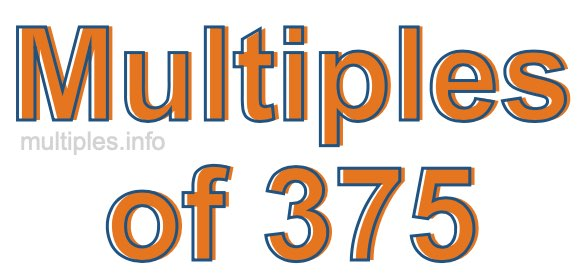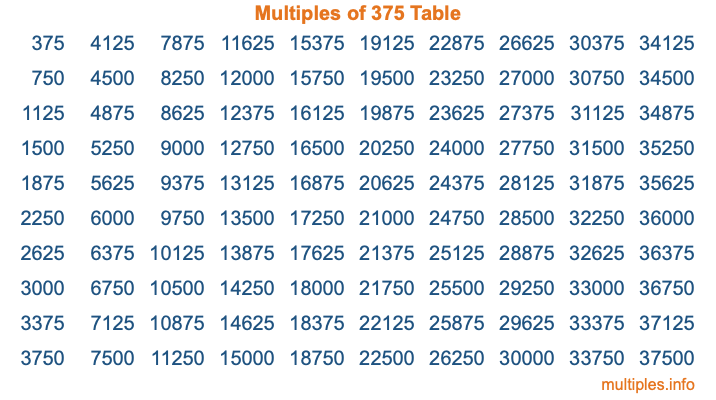Multiples of 375Welcome to the Multiples of 375 page. Here we will first teach you everything you will ever need to know about the multiples of 375, and then give you a study guide summary of everything we taught you to make sure you remember it all. Use this page to look up facts and learn information about the multiples of 375. This page will make you a multiples of three hundred seventy-five expert!

Definition of Multiples of 375
Multiples of 375 are all the numbers that when divided by 375 equal an integer. Each of the multiples of 375 are called a multiple. A multiple of 375 is created by multiplying 375 by an integer.

Therefore, to create a list of multiples of 375, you start with 1 multiplied by 375, then 2 multiplied by 375, then 3 multiplied by 375, and so on for as long as you want. Thus, the list of the first five multiples of 375 is 375, 750, 1125, 1500, and 1875. To see a larger list of multiples of 375, see the printable image of Multiples of 375 further down on this page. We also have a category where you can choose any nth multiple of 375.

Multiples of 375 Checker
The Multiples of 375 Checker below checks to see if any number of your choice is a multiple of 375. In other words, it checks to see if there is any number (integer) that when multiplied by 375 will equal your number. To do that, we divide your number by 375. If the the quotient is an integer, then your number is a multiple of 375.

Is  a multiple of 375?

Least Common Multiple of 375 and ...
A Least Common Multiple (LCM) is the lowest multiple that two or more numbers have in common. This is also called the smallest common multiple or lowest common multiple and is useful to know when you are adding our subtracting fractions. Enter one or more numbers below (375 is already entered) to find the LCM.

Check out our LCM Calculator if you need more details about the Least Common Multiple or if you need the LCM for different numbers for adding and subtraction fractions.

nth Multiple of 375
As we stated above, 375 is the first multiple of 375, 750 is the second multiple of 375, 1125 is the third multiple of 375, and so on. Enter a number below to find the nth multiple of 375.

th multiple of 375

Multiples of 375 vs Factors of 375
375 is a multiple of 375 and a factor of 375, but that is where the similarities end. All postive multiples of 375 are 375 or greater than 375. All positive factors of 375 are 375 or less than 375.

Below is the beginning list of multiples of 375 and the factors of 375 so you can compare:

Multiples of 375: 375, 750, 1125, 1500, 1875, etc.

Factors of 375: 1, 3, 5, 15, 25, 75, 125, 375

As you can see, the multiples of 375 are all the numbers that you can divide by 375 to get a whole number. The factors of 375, on the other hand, are all the whole numbers that you can multiply by another whole number to get 375.

It's also interesting to note that if a number (x) is a factor of 375, then 375 will also be a multiple of that number (x).

Multiples of 375 vs Divisors of 375
The divisors of 375 are all the integers that 375 can be divided by evenly. Below is a list of the divisors of 375.

Divisors of 375: 1, 3, 5, 15, 25, 75, 125, 375

The interesting thing to note here is that if you take any multiple of 375 and divide it by a divisor of 375, you will see that the quotient is an integer.

Multiples of 375 Table
Below is an image of the first 100 multiples of 375 in a table. The table is in chronological order, column by column. The first column has the first ten multiples of 375, the second column has the next ten multiples of 375, and so on.The Multiples of 375 Table is also referred to as the 375 Times Table or Times Table of 375. You are welcome to print out our table for your studies.

Negative Multiples of 375
Although not often discussed or needed in math, it is worth mentioning that you can make a list of negative multiples of 375 by multiplying 375 by -1, then by -2, then by -3, and so on, to get the following list of negative multiples of 375:

-375, -750, -1125, -1500, -1875, etc.

Multiples of 375 Summary
Below is a summary of important Multiples of 375 facts that we have discussed on this page. To retain the knowledge on this page, we recommend that you read through the summary and explain to yourself or a study partner why they hold true.

There are an infinite number of multiples of 375.

A multiple of 375 divided by 375 will equal a whole number.

375 divided by a factor of 375 equals a divisor of 375.

The nth multiple of 375 is n times 375.

The largest factor of 375 is equal to the first positive multiple of 375.

375 is a multiple of every factor of 375.

375 is a multiple of 375.

A multiple of 375 divided by a divisor of 375 equals an integer.

375 divided by a divisor of 375 equals a factor of 375.

Any integer times 375 will equal a multiple of 375.

Multiples of a Number
Here you can get the multiples of another number, all with the same attention to detail as we did for multiples of 375 on this page.

Multiples of
Multiples of 376
Did you find our page about multiples of three hundred seventy-five educational? Do you want more knowledge? Check out the multiples of the next number on our list!